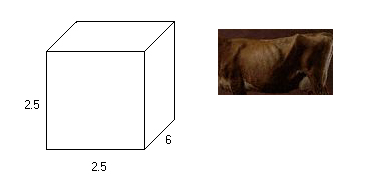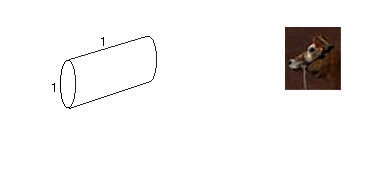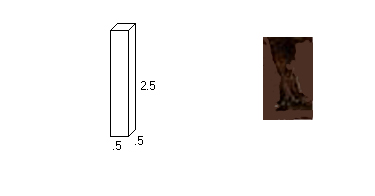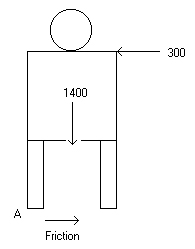# The Statics of Cow Tipping

The idea behind cow tipping is based on the belief that cows sleep standing up. This means that a person could walk right up next to the cow while it was standing perfectly still. This belief started the prank of cow tipping, where an individual would go up next to a cow that was sleeping and attempt to push it over on it's side. Why a person would do this is uncertain, but the fact that many people have tried it remains. From everything that I personally have heard, it is impossible for a single person to tip a cow on its side. The following is an in depth look at whether or not this prank is possible when several assumptions are made and figures are approximated.## Approximate cow part sizes (feet)

(Source: Local Farmers)

## BodyVolume = (2.5)(2.5)(6) = 37.5Volume = (3.14)(.5)(.5)(1) = .785

## Legs x 4Volume = (.5)(.5)(2.5) = .625

## Volume = Negligable## Center of Mass

Part Volume XCenter YCenter ZCenter X(V) Y(V) Z(V)
Body 37.5 1.25 3 3.75 46.875 112.5 140.625
Head .785 1.25 6.5 5.5 .98125 5.1025 4.3175
Leg 1 .625 .25 .25 1.25 .15625 .15625 .78125
Leg 2 .625 2.25 .25 1.25 1.40625 .15625 .78125
Leg 3 .625 .25 2.25 1.25 .15625 1.40625 .78125
Leg 4 .625 2.25 2.25 1.25 1.40625 1.40625 .78125
Total 40.785 ---- ---- ---- 50.98125 120.7275 148.0675

XCenter = 50.98125 / 40.785 = 1.25

YCenter = 120.7275 / 40.785 = 2.96

ZCenter = 148.0675 / 40.785 = 3.63Mass of average cow = 1400 lbs (Source: Local Farmers) Pushing force of human (Source: UNL Football Asst.) Average height of person = 6' Average height of arms = 5' Average horizontal push force = 300 lbs Force of Friction = 1400(.45) = 630 lbs (Assumed) (Cow will not slide)Back View of CowMA = 300(5) - 1400(1.25) = -250 (Counter-clockwise)# Results

After taken a counter-clockwise moment about the point A, it is shown that the weight of the cow creates more turning force than the human pushing force. The force of friction is not important here because it's force vector runs through the point A. The normal force can also be left out because before the pushing force is included the normal force of 1400 lbs. is divided in two with equal forces pushing at the center of both legs upward. This means that the cow is balanced. The normal force, though, moves to point A when the pushing force is applied, meaning that this force cancels out. The calculated moment comes out to negative 250 foot pounds, which can also be thought of as 250 foot pounds of force keeping the cows feet pressed against the ground. According to these calculations, it is impossible for an average person to tip an average cow by them self.# How many people to tip the cow?

### Try 2 people

Pushing force = 2 x 300 = 600 lbs
MA = 600(5) - 1400(1.25) = 1250
(Counter-clockwise)# Conclusion

Since it has already been shown that one person cannot tip a cow by them self, we can figure out just how many people would have to be pushing in order to complete the prank. By adding the second person, the amount of human pushing force is doubled to 600 pounds, still less than the force of friction. When the moment is calculated about A again, we find that there is now a positive 1250 foot pounds of force turning the cow about its feet and therefore tipping the cow on it's side. According to these numbers, cow tipping is possible when two people are in on the prank.